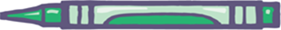# Fractions

## Objective

Measure lengths to the nearest quarter inch.

## Common Core Standards

### Core Standards

?

• 3.MD.B.4 — Generate measurement data by measuring lengths using rulers marked with halves and fourths of an inch. Show the data by making a line plot, where the horizontal scale is marked off in appropriate units— whole numbers, halves, or quarters.

?

• 2.MD.A.1

## Criteria for Success

?

1. Use the mark and move forward strategy to accurately create and label an inch ruler (spiral from Grade 2).
2. Partition an inch ruler so that each inch is partitioned into quarter inches.
3. Measure objects to the nearest quarter inch (MP.6).

## Tips for Teachers

?

• In the Problem Set, students will estimate the length of objects and then compare their estimate to the actual measurement. You could be very strategic and set out particular items that you’d like students to measure or students can find objects around the classroom on their own.
• In the Homework, students will estimate and then measure the length of various objects. Thus, have students bring home the same rulers that they used for the Problem Set.

#### Fishtank Plus

• Problem Set
• Student Handout Editor
• Vocabulary Package

?

### Problem 1

Wendell and Robin are trying to measure the length of various insects in their bug collection. The measurement of an ant is shown below.1. Wendell thinks the ant is about an inch long. Robin thinks the ant is closer to zero inches long. Do you agree with Wendell or Robin?
2. If you were to estimate a more exact measurement of the ant’s length, about how long would you say the ant is?
3. Use the same reasoning as Part (b) to approximate the length of the ladybug shown below.### Problem 2

Construct a quarter-inch ruler by doing the following:

1. Align an inch tile with one end of the strip of paper. Mark where the end of the inch tile is and label it with its corresponding length.
2. Move the inch tile to align with the mark you just drew. Label your mark with its corresponding length. Repeat this step until you reach the end of the ruler.
3. Fold the strip of paper so that each whole unit is partitioned into four equal parts.

### Problem 3

Measure the following objects with your ruler.

a.b.c.d.#### References

EngageNY Mathematics Grade 2 Mathematics > Module 7 > End-of-Module Assessment TaskQuestion #1

Grade 2 Mathematics > Module 7 > End-of-Module Assessment Task of the New York State Common Core Mathematics Curriculum from EngageNY and Great Minds. © 2015 Great Minds. Licensed by EngageNY of the New York State Education Department under the CC BY-NC-SA 3.0 US license. Accessed April 2, 2019, 3:52 p.m..

Modified by The Match Foundation, Inc.

## Problem Set & Homework

#### Discussion of Problem Set

• How did you determine the length of the ribbon in #3?
• If you were asked to find the length of the line in #4 to the nearest quarter-inch, how would your answer change? How did you determine the length of the line to the nearest half inch, since its length isn’t particularly close to a half inch?
• In what ways did measuring the bugs in #5 require even more attention to precision than usual?
• How did you determine the length of the pencil in #6? What made it more difficult than finding the length of the nail in #3?

?

### Problem 1

Brandi measures the length of the lizard as shown.What is the length of the lizard?

A.  $3\frac{1}{4}$ inches

B.  $3\frac{1}{2}$ inches

C.  $3\frac{3}{4}$ inches

D.  $4$ inches

#### References

PARCC Released Items Math Spring 2017 Grade 3 Released ItemsQuestion #36

### Problem 2

Measure the length of the line below to the nearest quarter inch.#### References

Achievement First Grade 3, Unit 6, Lesson 1

Grade 3, Unit 6, Lesson 1 is made available by Achievement First as a part of their Open Source web portal under a CC BY 4.0 license. Copyright © 1999-2017 Achievement First. Accessed April 2, 2019, 3:56 p.m..

Modified by The Match Foundation, Inc.

?